# Useful Fourier Transform-Pairs

Use the standard functions in Table 4.1 to form test signals, data patterns, and pulses , or to feather the edges of fast-changing signals.

The first three entries reveal the DFT sequences necessary to create time-domain pulses and clock waveforms. If the pulse width in each case is set to an integral number of time samples, the time-domain transform will display no aliasing. That's nice for creating good-looking charts and graphs, but doesn't really hide the aliasing problem. If you shift the waveform by a nonintegral number of sample points (using the shift operator), the aliasing, also called Gibbs phenomenon , comes back. The only true solution to aliasing is to apply somewhere within your simulation a heavy-duty smoothing filter and sample on a dense grid of points with a spacing at least 4x finer than the risetime of the smoothing filter. If nothing else, you should be simulating the risetime of your oscilloscope probe or the bandwidth of your receiver using a Gaussian filter.

The next three entries depict various edge-smoothing filters. The linear filter leaves a square-edged signal with an unrealistically large amount of high-frequency content. The high-frequency content generates aliasing that must be fixed by either (1) further filtering the signal to limit its bandwidth, or (2) using an outrageously high sampling rate.

For general work I use the Gaussian filter the most. Provided the Gaussian risetime is set to a minimum of four sample points, it practically eliminates aliasing.

The quadratic filter lies between the extremes of the linear and Gaussian filters. If used as the only band -limiting filter in a simulation, it requires a sampling rate greater than the Gaussian filter but not as great as the linear-ramp filter. Quadratic responses are popular with some IBIS model proponents because the time-domain result is parabolic and therefore easy to compute. This filter synthesizes the same exact parabolic response.

If you must simulate ramp waveforms in your circuit, then I suggest you use the linear-ramp filter with the necessary risetime followed by a Gaussian filter with a much smaller (10x smaller) risetime, and then sample the composite signal on a dense grid 4x finer than the Gaussian filter risetime.

Table 4.1. Useful Fourier Transform Pairs

Fourier Transform

DFT

Pulse of width b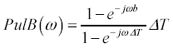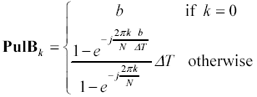Pulse of width ( N /2) D T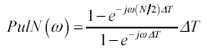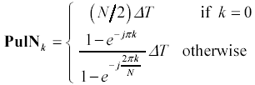Clock waveform with M complete cycles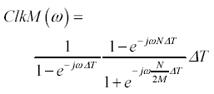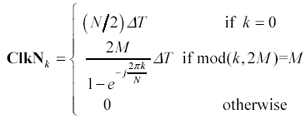Delay operator (delays by amount t )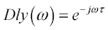Linear-ramp LPF with 10-90% rise-fall time r (set q = 1.25 · r )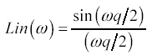Quadratic LPF with 10% to 90% rise/fall time r (set q = 0.9045084972 · r )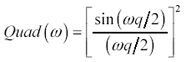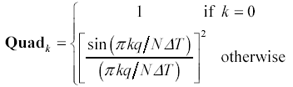Gaussian LPF with 10% to 90% rise/fall time r (set q = .275 · r )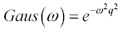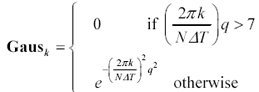POINT TO REMEMBER

• Table 4.1 shows how to form FFT frequency vectors for test signals, data patterns, pulses, and feathered edges.High-Speed Signal Propagation[c] Advanced Black Magic
ISBN: 013084408X
EAN: N/A
Year: 2005
Pages: 163